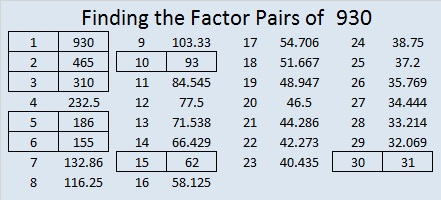# 930 Witch’s Broom

This witch’s broom is a puzzle based on the multiplication table. The factors from 1 to 12 are out of order in the first column and the top row, but they all belong somewhere in each place. Use logic to figure out where those factors go. It’s a level 6, so it will be a little tricky, but not as tricky as it sometimes is. Solve it, and you might feel like flying!Print the puzzles or type the solution on this excel file: 12 factors 923-931

What is special about the number 930?

463 + 467 = 930, so it is the sum of consecutive prime numbers.

30 × 31 = 930 The factors in that factor pair are consecutive so 930 is the sum of the first 30 EVEN numbers:
2 + 4 + 6 + 8 + . . . + 54 + 56 + 58 + 60 = 930

930 is the hypotenuse of a Pythagorean triple:
558-744-930, which is (3-4-5) times 186.

Here’s 930 in a few other bases:
Palindrome 656 in BASE 12, because 6(144) + 5(12) + 6(1) = 930
110 in BASE 30, because 1(30²) + 1(30) + 0(1) = 30(31) = 930
U0 in BASE 31 (U is 30 base 10), because 30(31) + 0(1) = 930

• 930 is a composite number.
• Prime factorization: 930 = 2 × 3 × 5 × 31
• The exponents in the prime factorization are 1, 1, 1, and 1. Adding one to each and multiplying we get (1 + 1)(1 + 1)(1 + 1)(1 + 1) = 2 × 2 × 2 × 2 = 16. Therefore 930 has exactly 16 factors.
• Factors of 930: 1, 2, 3, 5, 6, 10, 15, 30, 31, 62, 93, 155, 186, 310, 465, 930
• Factor pairs: 930 = 1 × 930, 2 × 465, 3 × 310, 5 × 186, 6 × 155, 10 × 93, 15 × 62, or 30 × 31
• 930 has no square factors that allow its square root to be simplified. √930 ≈ 30.495901.